### Home > AC > Chapter 10 > Lesson 10.3.2 > Problem10-116

10-116.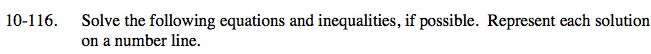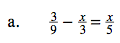Multiply the equation by a common multiple of the denominators to remove the fractions.

$45\left(\frac{3}{9}-\frac{x}{3}=\frac{x}{5}\right)$

15 − 15x = 9x

Solve for x.

$\frac{5}{8} = x$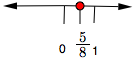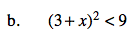Find the boundary point.
(3 + x)² = 9

Take the square root of both sides.
3 + x = ± 3

Solve for x.

Test numbers on all sides of the boundary points.

(3 + (−7))2 < 9 (3 + (−1))2 < 9 (3 + (1))2 < 9
(−4)2 < 9 22 < 9 42 < 9
16 < 9 4 < 9 16 < 9
False True False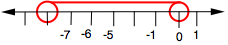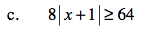Divide both sides by 8.

The absolute value of what two numbers equals 8?

Follow the steps in part (b).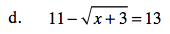Subtract 11 from both sides.

Square both sides.

Subtract 3 from both sides.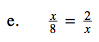Follow the steps in part (a).

A common multiple of x and 8 is 8x.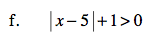Follow the steps in part (c).